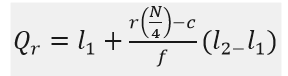Checkout JEE MAINS 2022 Question Paper Analysis : Checkout JEE MAINS 2022 Question Paper Analysis :

# Quartiles

In statistics, Quartiles are the set of values which has three points dividing the data set into four identical parts. We ordinarily deal with a large amount of numerical data, in stats. There are several concepts and formulas, which are extensively applicable in various researches and surveys.  One of the best applications of quartiles is defined in box and whisker plot.

Quartiles are the values that divide a list of numerical data into three quarters. The middle part of the three quarters measures the central point of distribution and shows the data which are near to the central point. The lower part of the quarters indicates just half information set which comes under the median and the upper part shows the remaining half, which falls over the median. In all, the quartiles depict the distribution or dispersion of the data set.

## Quartiles Definition

Quartiles divide the entire set into four equal parts. So, there are three quartiles, first, second and third represented by Q1, Q2 and Q3, respectively. Q2 is nothing but the median, since it indicates the position of the item in the list and thus, is a positional average. To find quartiles of a group of data, we have to arrange the data in ascending order.

In the median, we can measure the distribution with the help of lesser and higher quartile. Apart from mean and median, there are other measures in statistics, which can divide the data into specific equal parts. A median divides a series into two equal parts. We can partition values of a data set mainly into three different ways:

1. Quartiles
2. Deciles
3. Percentiles

### Quartiles Formula

Suppose, Q3 is the upper quartile is the median of the upper half of the data set. Whereas, Q1 is the lower quartile and median of the lower half of the data set. Q2 is the median. Consider, we have n number of items in a data set. Then the quartiles are given by;

Q1 = [(n+1)/4]th item

Q2 = [(n+1)/2]th item

Q3 = [3(n+1)/4]th item

Hence, the formula for quartile can be given by;Where, Qr is the rth quartile

l1 is the lower limit

l2 is the upper limit

f is the frequency

c is the cumulative frequency of the class preceding the quartile class.

### Quartiles in Statistics

Similar to the median which divides the data into half so that 50% of the estimation lies below the median and 50% lies above it, the quartile splits the data into quarters so that 25% of the estimation are less than the lower quartile, 50% of estimation are less than the mean, and 75% of estimation are less than the upper quartile. Usually, the data is ordered from smallest to largest:

• First quartile: 25% from smallest to largest of numbers
• Second quartile: between 25.1% and 50% (till median)
• Third quartile: 51% to 75% (above the median)
• Fourth quartile: 25% of largest numbers

### Quartile Deviation

You have learned about standard deviation in statistics. Quartile deviation is defined as half of the distance between the third and the first quartile. It is also called Semi Interquartile range. If Q1 is the first quartile and Q3 is the third quartile, then the formula for deviation is given by;

 Quartile deviation = (Q3-Q1)/2

### Interquartile Range

The interquartile range (IQR) is the difference between the upper and lower quartile of a given data set and is also called a midspread. It is a measure of statistical distribution, which is equal to the difference between the upper and lower quartiles. Also, it is a calculation of variation while dividing a data set into quartiles. If Q1 is the first quartile and Q3 is the third quartile, then the IQR formula is given by;

 IQR = Q3 – Q1

### Quartiles Examples

Question 1: Find the quartiles of the following data: 4, 6, 7, 8, 10, 23, 34.

Solution: Here the numbers are arranged in the ascending order and number of items, n = 7

Lower quartile, Q1 = [(n+1)/4] th item

Q1= 7+1/4 = 2nd item = 6

Median, Q2 = [(n+1)/2]th item

Q2= 7+1/2 item = 4th item = 8

Upper Quartile, Q3 = [3(n+1)/4]th item

Q3 = 3(7+1)/4 item = 6th item = 23

Question 2: Find the Quartiles of the following age:-

23, 13, 37, 16, 26, 35, 26, 35

Solution:

First, we need to arrange the numbers in increasing order.

Therefore, 13, 16, 23, 26, 26, 35, 35, 37

Number of items, n = 8

Lower quartile, Q1 = [(n+1)/4] th item

Q1 = 8+1/4 = 9/4 = 2.25th term

From the quartile formula we can write;

Q1 = 2nd term + 0.25(3rd term-2nd term)

Q1= 16+0.25(23-26) = 15.25

Similarly,

Median, Q2 = [(n+1)/2]th item

Q2 = 8+1/2 = 9/2 = 4.5

Q2 = 4th term+0.5 (5th term-4th term)

Q2= 26+0.5(26-26) = 26

And,

Upper Quartile, Q3 = [3(n+1)/4]th item

Q3 = 3(8+1)/4 = 6.75th term

Q3 = 6th term + 0.75(7th term-6th term)

Q3 = 35+0.75(35-35) = 35